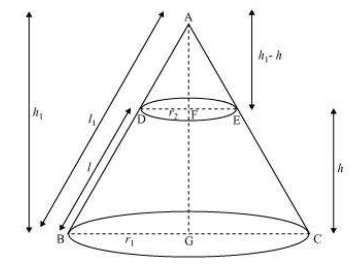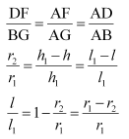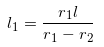Newbie

# Derive the formula for the curved surface area and total surface area of the frustum of a cone, given to you in Section 13.5, using the symbols as explained. Q.6

• 0

Today i am solving the surface areas and volumes of ncert class 10th of exercise 13.5 question number 6. Find the best solution of this question  give me the easiest solution of this question .,also find the simplest and best solution of this question.

Share

1. Consider the diagramLet ABC be a cone. From the cone the frustum DECB is cut by a plane parallel to its base. Here, r1 and r2 are the radii of the frustum ends of the cone and h be the frustum height.

Now, consider the ΔABG and ΔADF,

Here, DF||BGNow, by rearranging we get,The total surface area of frustum will be equal to the total CSA of frustum + the area of upper circular end + area of the lower circular end

= π(r1+r2)l+πr22+πr12

∴ Surface area of frustum = π[r1+r2)l+r12+r22]

• 0